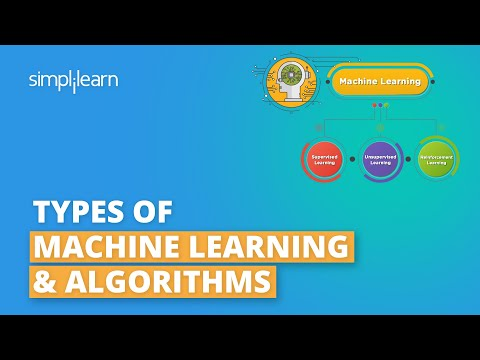Machine Learning has found its applications in almost every business sector. There are several algorithms used in machine learning that help you build complex models. Each of these algorithms in machine learning can be classified into a certain category. In this article, we’ll learn about the types of machine learning. This will give you better insight into the field of machine learning.

## What is Machine Learning?

Machine Learning is an application of Artificial Intelligence that enables systems to learn from vast volumes of data and solve specific problems. It uses computer algorithms that improve their efficiency automatically through experience.There are primarily three types of machine learning: Supervised, Unsupervised, and Reinforcement Learning.Let’s explore and understand the different types of machine learning one by one.

#### Machine Learning FREE Course

Take the 1st Step to Machine Learning Success## Supervised Learning

### Overview:

Supervised learning is a type of machine learning that uses labeled data to train machine learning models. In labeled data, the output is already known. The model just needs to map the inputs to the respective outputs.

An example of supervised learning is to train a system that identifies the image of an animal.

Attached below, you can see that we have our trained model that identifies the picture of a cat.### Algorithms:

Some of the most popularly used supervised learning algorithms are:

• Linear Regression
• Logistic Regression
• Support Vector Machine
• K Nearest Neighbor
• Decision Tree
• Random Forest
• Naive Bayes

### Working:

Supervised learning algorithms take labeled inputs and map them to the known outputs, which means you already know the target variable.

Now, let’s focus on the training process for the supervised learning method.

Supervised Learning methods need external supervision to train machine learning models. Hence, the name supervised. They need guidance and additional information to return the desired result.

### Applications:

Supervised learning algorithms are generally used for solving classification and regression problems.Few of the top supervised learning applications are weather prediction, sales forecasting, stock price analysis.Now that you understand what Supervised learning is, let’s see the next type of machine learning.

## Unsupervised Learning

### Overview:

Unsupervised learning is a type of machine learning that uses unlabeled data to train machines. Unlabeled data doesn’t have a fixed output variable. The model learns from the data, discovers the patterns and features in the data, and returns the output.

Depicted below is an example of an unsupervised learning technique that uses the images of vehicles to classify if it’s a bus or a truck. The model learns by identifying the parts of a vehicle, such as a length and width of the vehicle, the front, and rear end covers, roof hoods, the types of wheels used, etc. Based on these features, the model classifies if the vehicle is a bus or a truck.### Algorithms:

Selecting the right algorithm depends on the type of problem you are trying to solve. Some of the common examples of unsupervised learning are:

• K Means Clusterin
• Hierarchical Clustering
• DBSCAN
• Principal Component Analysis

### Working:

Unsupervised learning finds patterns and understands the trends in the data to discover the output. So, the model tries to label the data based on the features of the input data.

The training process used in unsupervised learning techniques does not need any supervision to build models. They learn on their own and predict the output.

### Applications:

Unsupervised learning is used for solving clustering and association problems.One of the applications of unsupervised learning is customer segmentation. Based on customer behavior, likes, dislikes, and interests, you can segment and cluster similar customers into a group. Another example where unsupervised learning algorithms are used is used churn rate analysis.Let’s see the third type of machine learning, i.e., reinforcement learning.

## Reinforcement Learning

### Overview

Reinforcement Learning trains a machine to take suitable actions and maximize its rewards in a particular situation. It uses an agent and an environment to produce actions and rewards. The agent has a start and an end state. But, there might be different paths for reaching the end state, like a maze. In this learning technique, there is no predefined target variable.

An example of reinforcement learning is to train a machine that can identify the shape of an object, given a list of different objects. In the example shown, the model tries to predict the shape of the object, which is a square in this case.### Algorithms

Some of the important reinforcement learning algorithms are:

1. Q-learning
2. Sarsa
3. Monte Carlo
4. Deep Q network

### Working

Reinforcement learning follows trial and error methods to get the desired result. After accomplishing a task, the agent receives an award. An example could be to train a dog to catch the ball. If the dog learns to catch a ball, you give it a reward, such as a biscuit.

Reinforcement Learning methods do not need any external supervision to train models.

Reinforcement learning problems are reward-based. For every task or for every step completed, there will be a reward received by the agent. If the task is not achieved correctly, there will be some penalty added.Now, let’s see what applications we have in reinforcement learning.

### Applications

Reinforcement learning algorithms are widely used in the gaming industries to build games. It is also used to train robots to do human tasks.Acelerate your career in AI and ML with the Post Graduate Program in AI and Machine Learning with Purdue University collaborated with IBM.

## Conclusion

After reading this article, you would have learned the basics of machine learning and its different types. You would have understood the training process and the types of problems they solve. Finally, you looked at the various applications of machine learning.Kartik Menon